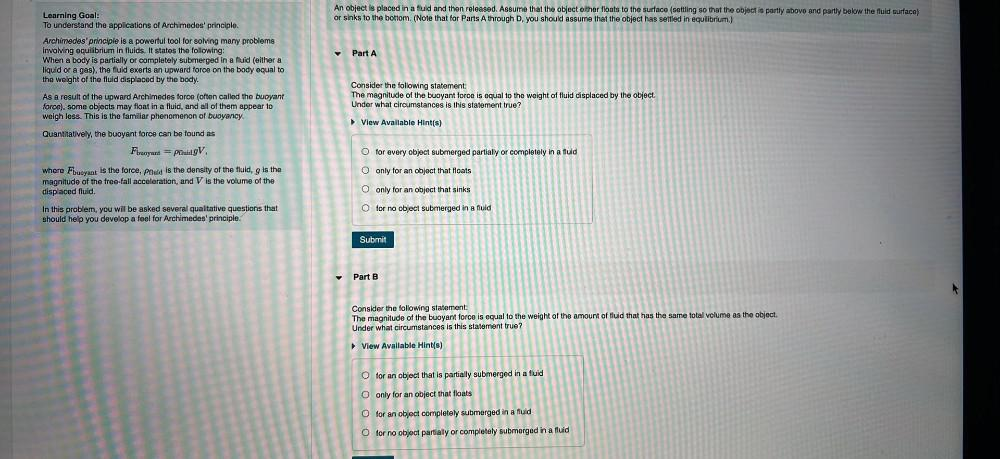Question:

# An object is placed in a fluid and then released. Assume that the object either floats to the surface (settling so that the objeAn object is placed in a fluid and then released. Assume that the object either floats to the surface (settling so that the object is partly above and partly below the fluid surface) or sinks to the bottom. (Note that for Parts A through D. you should assume that the object has settled in equilibrium.) Learning Goal: To understand the applications of Archimedes' principle. Archimedes' principle is a powerful tool for solving many problems involving equilibrium in fluids. It states the following: When a body is partially or completely submerged in a fluid (either a liquid or a gas), the fluid exerts an upward force on the body equal to the weight of the fluid displaced by the body. Part A As a result of the upward Archimedes force (often called the buoyant force), some objects may float in a fluid, and all of them appear to weigh less. This is the familiar phenomenon of buoyancy. Consider the following statement: The magnitude of the buoyant force is equal to the weight of fluid displaced by the object. Under what circumstances is this statement true? View Available Hint(s) Quantitatively, the buoyant force can be found as O for every object submerged partially or completely in a fluid Fbuoyant = pfluid 9V where Fbuoyant is the force, Pfluid is the density of the fluid, g is the magnitude of the free-fall acceleration, and V is the volume of the displaced fluid. O only for an object that floats O only for an object that sinks O for no object submerged in a fluid In this problem, you will be asked several qualitative questions that should help you develop a feel for Archimedes' prin Submit Part B Consider the following statement: The magnitude of the buoyant force is equal to the weight of the amount of fluid that has the same total volume as the object. Under what circumstances is this statement true? ► View Available Hint(s) O for an object that is partially submerged in a fluid O only for an object that floats O for an object completely submerged in a fluid O for no object partially or completely submerged in a fluid